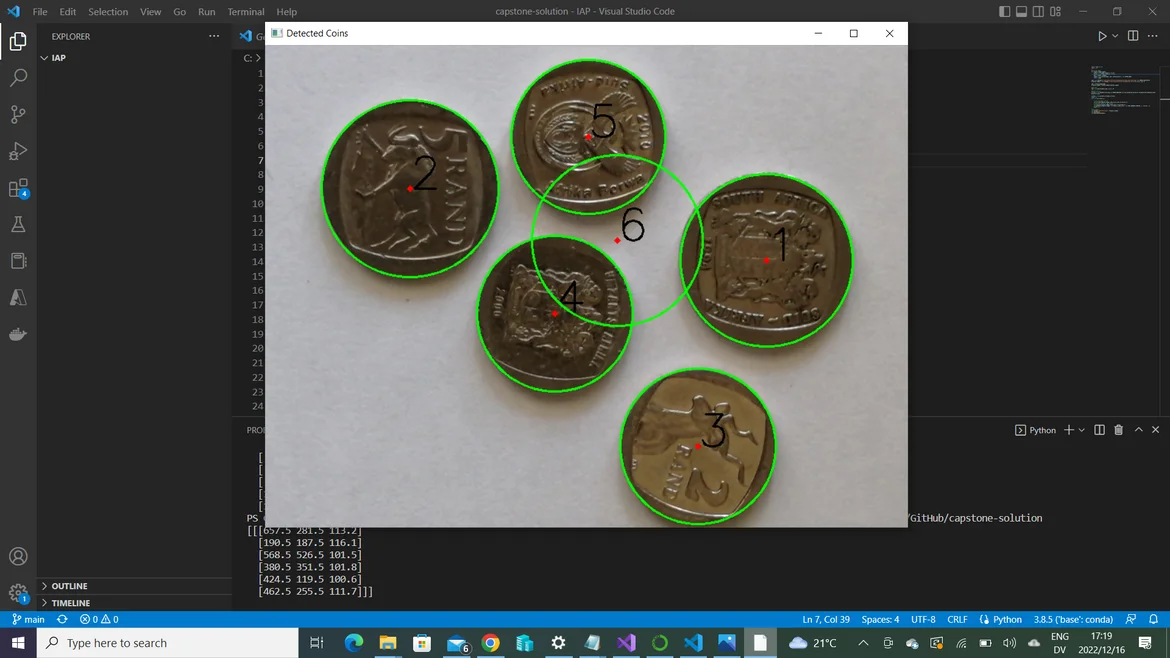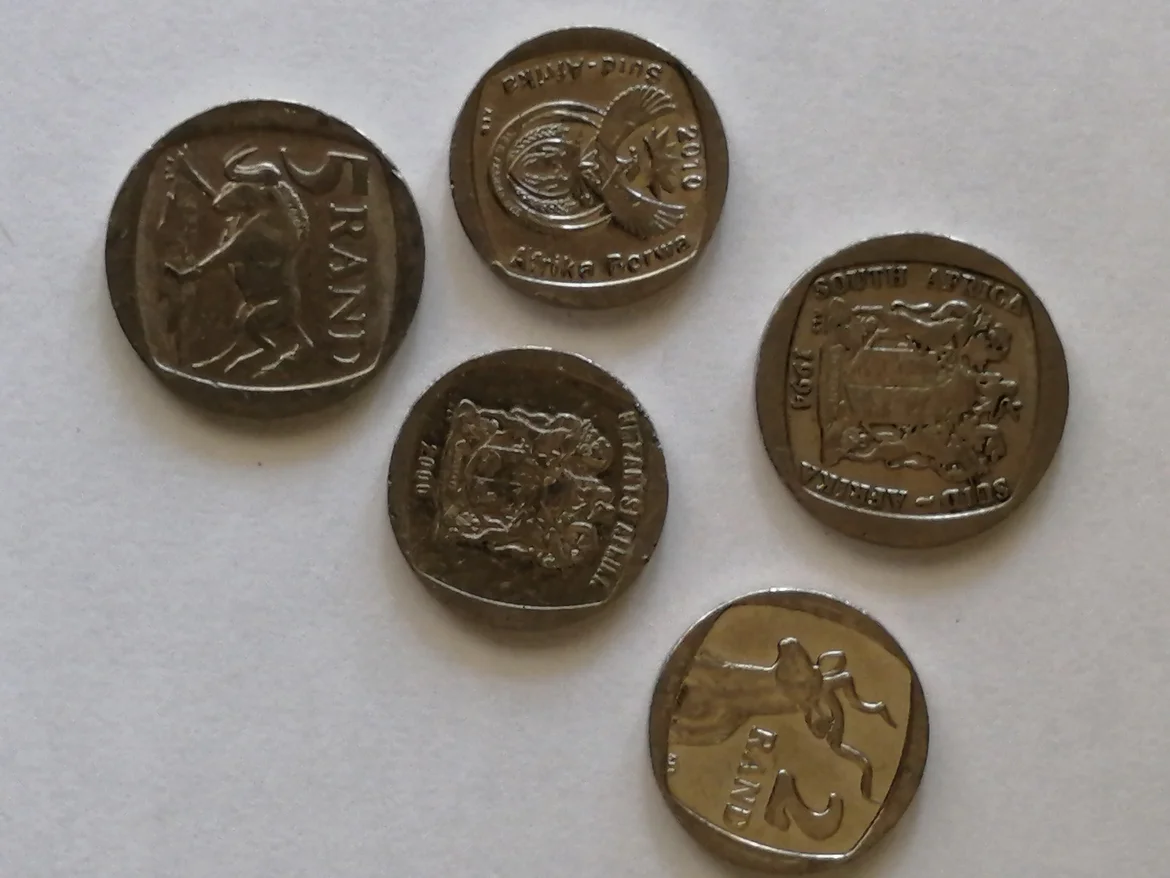18 Dec 2022

Posted on:

16 Dec 2022

0

# Resolved:Imaginary circles detected

My program is detecting circles that are not there as well as the coins. How do I fix this?

Here is my code:

``````import numpy as np
import cv2

#resizing image
def resize_image(image):
width = int(image.shape * 0.15)
height = int(image.shape * 0.15)
dim = (width, height)
image = cv2.resize(image, dim, interpolation = cv2.INTER_AREA)
return image

img = resize_image(img)
original_image = resize_image(original_image)

img = cv2.GaussianBlur(img, (5,5), 0)

#detect circles
print(circles)

circles = np.uint16(np.around(circles))
count = 1
for i in circles[0,:]:

# draw the outer circle
cv2.circle(original_image,(i,i),i,(0,255,0),2)
# draw the center of the circle
cv2.circle(original_image,(i,i),2,(0,0,255),3)
cv2.putText(original_image, str(count),(i,i), cv2.FONT_HERSHEY_SIMPLEX, 2, (0,0,0), 2)
count += 1

#show images
cv2.imshow('Detected Coins', original_image)
cv2.waitKey(0)
cv2.destroyAllWindows()
``````Posted on:

16 Dec 2022

0

Hey Quinn,

Thank you for reaching out!

Since you are using a different image than the one provided in the lecture, I can't reproduce the issue you're experiencing. It's possible that the problem stems from the function you've defined, namely `resize_image()`. Have you tried running the program without resizing the image?

Kind regards,
365 Hristina

Posted on:

16 Dec 2022

0

Hi Hristina,

Thank you for your suggestion. It results in 20 circles being detected though, so the sizing seems to be necessary. Below, I will attach the file that I am using, if that helps.Posted on:

18 Dec 2022

0

After combing through the documentation and StackOverflow carefully, I realised the problem lay in the values I inserted as arguments for the HoughCircles function. After tweaking those, the problem resolved